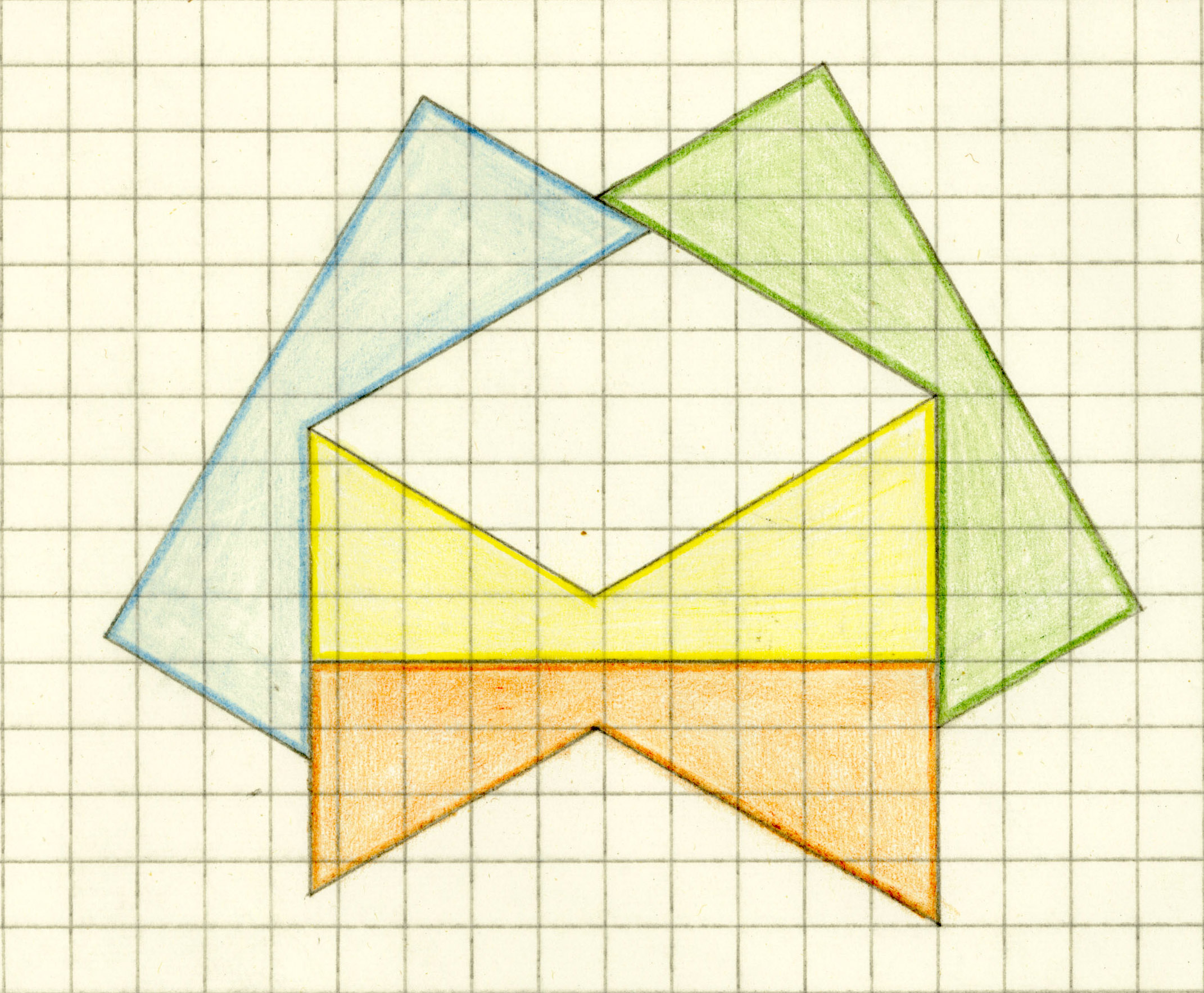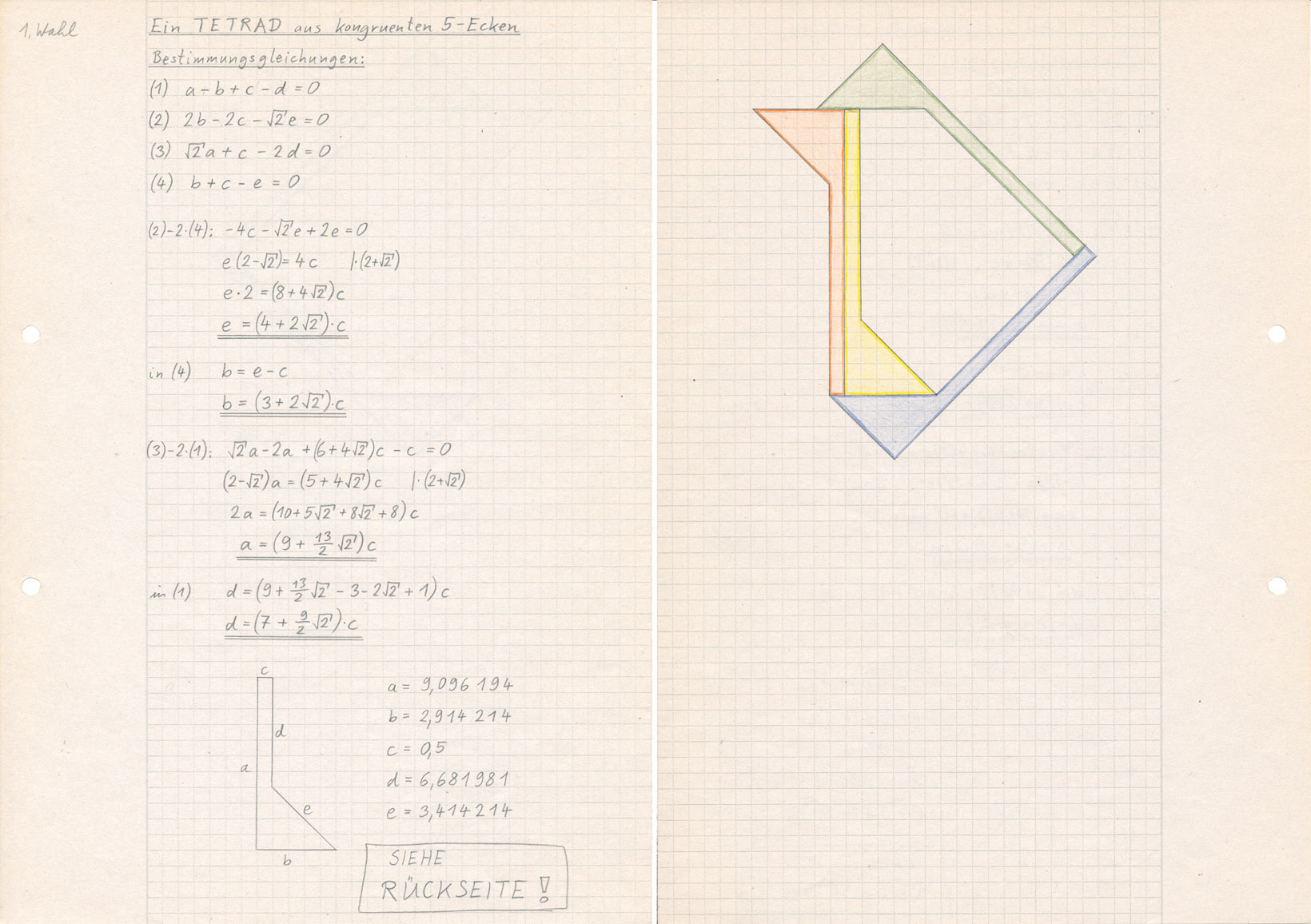14. Can four pentagons form a tetrad?

Yes, tetrads with a hole are possible. It is extremely unlikely that such a tetrad without hole exists, but I have not seen a proof.

(A) Here is my oldest tetrad made of pentagons. It was also sent to Martin Gardner in 1979, but is not mentioned in his book.
It is very easy to construct with the angles 90°, 60° and 120°.
I cannot believe that this tetrad was not published anywhere in the last century.The old tetrad is already optimised because the critical three distances f, g and h are equal with maximum relative length.The number of vertices of the tetrad outline (including holes) is VT = 10 + 4 = 14.

(B) The next tetrad was invented by Livio Zucca. It is shown as a png-graphic on Georg Sicherman's website. 
This tetrad is considered as the first published tetrad with pentagon tiles. A svg-graphic of Livio Zucca's tetrad is shown as first graphic below.

In my opinion the quality of a polygon tetrad is higher if its outline (including holes) has a smaller number VT of vertices.
It is possible to reduce this number by 2. VT = 8 + 5 = 13. Moreover the minimum length of common borders is b. The modified tetrad shown as second graphic below is an octagon and its hole is a pentagon.
But now the leftmost vertex of the green tile is near an edge of the yellow tile. And vertices of the red and blue tile are nearer to vertices of the green tile. In fact an objective criterion for the quality of a tetrad does not exist.(C) A new (January 2020) tetrad with pentagon tiles and VT = 9 + 4 = 13.
Here properties of the old tetrad and Livio Lucca's tetrad are combined. Increasing the common border between the red and the blue tile would decrease the distance between the vertex with the largest internal angle and the opposite edge.(D) An old tetrad (1980 - 1990) with pentagon tiles and VT = 7 + 5 = 12.(E) A new (February 2020) tetrad with pentagon tiles and VT = 7 + 4 = 11.
With the same principles applied in (C) the tetrad (D) was modified.
The tetrad is a heptagon with an obtuse trapezoid hole.As free parameters for the construction you can use the length b and the angle alpha.
A good choice is alpha = 55.754°, then the gap h has its maximum width.Open problem: Is there a tetrad made of four pentagons with VT smaller than 11?

 Previous Question Tetrads Contents Next Question